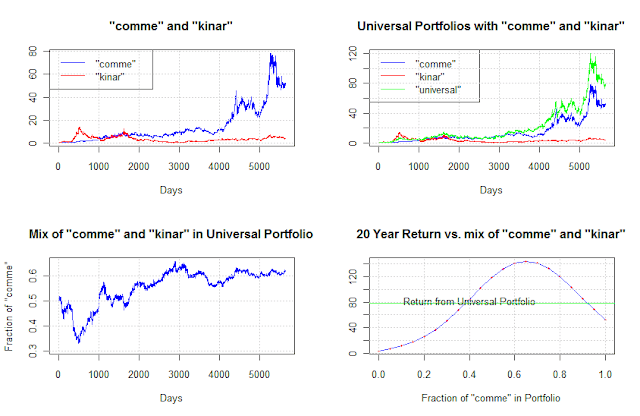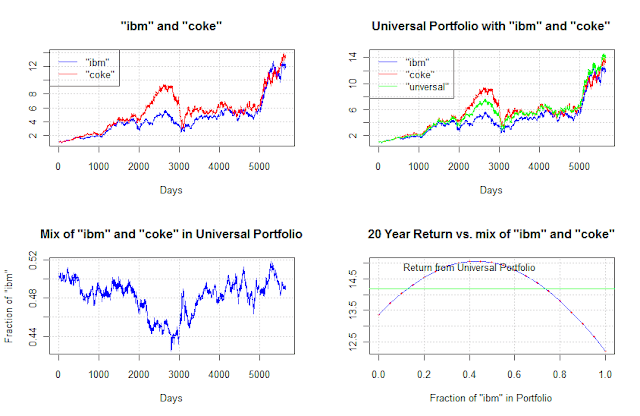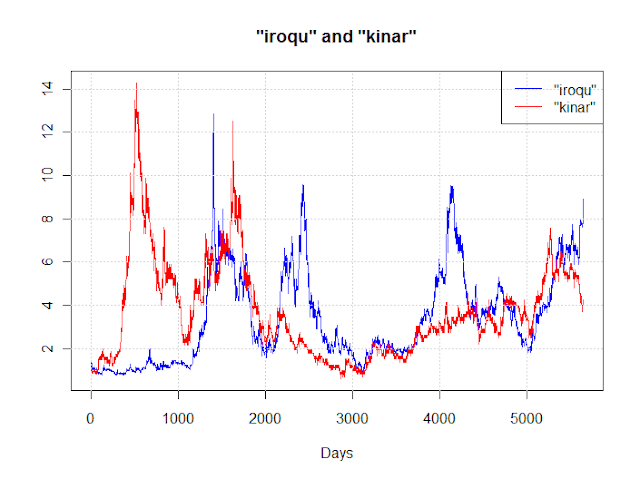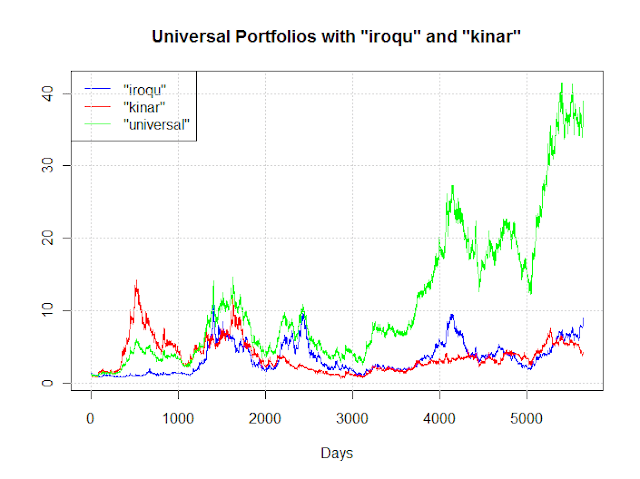## Saturday, June 30, 2012

### R is fun

As mentioned in Universal portfolio, part 6, the wealth reported in Table 8.4 of Universal Portfolios could not be reproduced.  An other observation is that the random weight vectors reported in Table 8.4 are shown in descending lexicographic order, except for the last one, suggesting possibly that there is an error in the original table.

R code allows to perform a simple experiment, take subsets of the full set of weights and check if we can reproduce the reported wealth.  Explicitly we'll try all subsets that exclude from 1 to 7 values in the original set.  This shows the expressive power of R, and how fast modern computers operate.  The whole search only takes a few line of codes and execute very fast even if this entails computing the mean of 280,599 different subsets.

As it turns out, two different subsets with 18 elements match the reported wealth, a surprising result.  No other subsets match.  The code below must be appended after the code used in Universal portfolio, part 6.

# remove selected entries to try to get the published value of 98.4240
# R is fun and modern computers are fast
BestAppr <- 0
nPruned <- 0
nC <- length(crps)
cat("\n")
for (m in seq(nC-1, nC-7)) {
CrpPruned <- combn(crps, m, mean)
nPruned <- nPruned + length(CrpPruned)
Best <- min(abs(CrpPruned - 98.4240))
cat(sprintf("Min delta from Cover with %d samples is %.4f\n", m, Best))
}
cat(sprintf("\n%d different subsets tried\n", nPruned))
cat("\n Subsets matching the wealth reported in Table 8.4\n")

# so at least one subset of 18 samples match the published value, show them
Indices <- combn(1:22,18)
for (i in 1:(dim(Indices))) {
PrunedMean <- mean(crps[Indices[,i]])
if (abs(PrunedMean - 98.4240) < 0.0001) {
cat(Indices[,i])
cat(sprintf("  %.5f\n",PrunedMean))
}
}

This gives the following output

Min delta from Cover with 21 samples is 2.3056
Min delta from Cover with 20 samples is 0.0398
Min delta from Cover with 19 samples is 0.0116
Min delta from Cover with 18 samples is 0.0000
Min delta from Cover with 17 samples is 0.0003
Min delta from Cover with 16 samples is 0.0008
Min delta from Cover with 15 samples is 0.0002

280599 different subsets tried

Subsets matching the wealth reported in Table 8.4

1 2 3 4 5 6 9 10 11 12 13 14 15 17 19 20 21 22  98.42403
1 2 3 4 5 6 9 11 12 13 14 15 17 18 19 20 21 22  98.42403

## Sunday, June 10, 2012

### Universal portfolio, part 6

The final table in Universal Portfolios introduces leverage.  It indirectly also shows the dangers of rebalancing on margin, while Kin Ark increases 4.2 times, at 50% margin it goes to nothing.

The code below reproduces Table 8.4, again as plain text only.  For unknown reason, the wealth of the universal portfolio cannot be reproduced, while all other values do match.

# table 8.4 of Cover "Universal Portfolios"
library(logopt)
x <- nyse.cover.1962.1984
xck <- x[,c("comme","kinar")]
nDays <- dim(xck)
Days <- 1:nDays

# calculate the margined sequence
r <- 0.000233 # seems to be r <- ((1+0.06) ^ (1/250)) - 1 rounded
yck <- (2 * xck) - 1 - r
xyck <- cbind(xck[,1], yck[,1], xck[,2], yck[,2])
mck <- cumprod(xyck)[nDays]

cat(sprintf("Commercial metals                       %.4f\n", mck[1,1]))
cat(sprintf("Commercial metals on margin             %.4f\n", mck[1,2]))
cat(sprintf("Kin Ark                                 %.4f\n", mck[1,3]))
cat(sprintf("Kin Ark on margin                       %.4f\n", mck[1,4]))
cat(sprintf("r = %.6f/day = 6%%/year\n",r))

# same "random" b as in Cover
b <-          c(0.8, 0.2, 0.0, 0.0)
b <- rbind(b, c(0.8, 0.1, 0.0, 0.1))
b <- rbind(b, c(0.6, 0.1, 0.1, 0.2))
b <- rbind(b, c(0.6, 0.0, 0.4, 0.0))
b <- rbind(b, c(0.5, 0.0, 0.2, 0.3))
b <- rbind(b, c(0.4, 0.0, 0.4, 0.2))
b <- rbind(b, c(0.3, 0.5, 0.1, 0.1))
b <- rbind(b, c(0.3, 0.4, 0.1, 0.2))
b <- rbind(b, c(0.3, 0.2, 0.2, 0.3))
b <- rbind(b, c(0.3, 0.1, 0.2, 0.4))
b <- rbind(b, c(0.3, 0.0, 0.1, 0.6))
b <- rbind(b, c(0.2, 0.7, 0.0, 0.1))
b <- rbind(b, c(0.2, 0.2, 0.3, 0.3))
b <- rbind(b, c(0.1, 0.8, 0.1, 0.0))
b <- rbind(b, c(0.1, 0.5, 0.2, 0.2))
b <- rbind(b, c(0.1, 0.4, 0.2, 0.3))
b <- rbind(b, c(0.1, 0.3, 0.1, 0.5))
b <- rbind(b, c(0.1, 0.2, 0.4, 0.3))
b <- rbind(b, c(0.1, 0.1, 0.2, 0.6))
b <- rbind(b, c(0.0, 0.5, 0.4, 0.1))
b <- rbind(b, c(0.0, 0.4, 0.2, 0.4))
b <- rbind(b, c(0.2, 0.5, 0.1, 0.2))

BestValue <- 0
crps <- b[,1] * 0
for (i in 1:length(crps)) {
crps[i] <- crp(xyck, b[i,])[nDays]
if(crps[i] > BestValue) {
BestValue <- crps[i]
BestB <- b[i,]
}
}

cat(sprintf("Sn* = %.4f                          bn* =(%.1f, %.1f, %.1f, %.1f)\n",
BestValue, BestB, BestB, BestB, BestB))
cat(sprintf("Best constituent stock                  %.4f\n",max(mck)))
cat(sprintf("Wealth achieved by universal portfolio  Sn^ = %.4f\n", mean(crps)))
cat(sprintf("\n         b                Sn(b)\n\n"))

for (i in 1:length(crps)) {
cat(sprintf("(%.1f, %.1f, %.1f %.1f)     %8.4f\n",b[i,1], b[i,2], b[i,3], b[i,4], crps[i]))
}

Giving the following output

Commercial metals                       52.0203
Commercial metals on margin             19.7335
Kin Ark                                 4.1276
Kin Ark on margin                       0.0000
r = 0.000233/day = 6%/year
Sn* = 262.4021                          bn* =(0.2, 0.5, 0.1, 0.2)
Best constituent stock                  52.0203
Wealth achieved by universal portfolio  Sn^ = 108.0784

b                Sn(b)

(0.8, 0.2, 0.0 0.0)      57.0535
(0.8, 0.1, 0.0 0.1)     148.9951
(0.6, 0.1, 0.1 0.2)     207.1143
(0.6, 0.0, 0.4 0.0)     140.7803
(0.5, 0.0, 0.2 0.3)      60.8358
(0.4, 0.0, 0.4 0.2)      47.6074
(0.3, 0.5, 0.1 0.1)     212.8928
(0.3, 0.4, 0.1 0.2)     261.0452
(0.3, 0.2, 0.2 0.3)      89.0330
(0.3, 0.1, 0.2 0.4)      19.4840
(0.3, 0.0, 0.1 0.6)       0.7700
(0.2, 0.7, 0.0 0.1)     121.0142
(0.2, 0.2, 0.3 0.3)      45.2562
(0.1, 0.8, 0.1 0.0)      67.5882
(0.1, 0.5, 0.2 0.2)     233.6328
(0.1, 0.4, 0.2 0.3)     112.6695
(0.1, 0.3, 0.1 0.5)      12.7702
(0.1, 0.2, 0.4 0.3)      19.4840
(0.1, 0.1, 0.2 0.6)       0.2354
(0.0, 0.5, 0.4 0.1)     225.2524
(0.0, 0.4, 0.2 0.4)      31.8076
(0.2, 0.5, 0.1 0.2)     262.4021

## Saturday, June 9, 2012

### Universal portfolio, part 5

The first three tables in Universal Portfolios presents the same information in numerical form as some of the plots.  The following code generates all three tables by defining a function then calling it with suitable parameters.  The output is text only, so does not match the typography of the original.

# tables 8.1, 8.2 and 8.3 of Cover "Universal Portfolios"
require(logopt)
x <- nyse.cover.1962.1984

ShowTable <- function(a,b) {
cat(sprintf("%s versus %s\n", a, b))
cat(sprintf("------------------\n"))
cat(sprintf("b            Sn(b)\n"))
cat(sprintf("------------------\n"))
xab <- x[,c(a,b)]
nDays <- dim(xab)
Days <- 1:nDays
alphas <- seq(0,1,by=0.05)
crps <- alphas
for (i in 1:length(crps)) {
crps[i] <- crp(xab, c(1-alphas[i], alphas[i]))[nDays]
cat(sprintf("%.2f     %9.4f\n",1-alphas[i], crps[i]))
}
cat(sprintf("------------------\n"))
cat(sprintf("Target wealth: Sn* = %.4f\n",max(crps)))
cat(sprintf("Best rebalanced portfolio: bn* = %4.2f\n",1-alphas[which.max(crps)]))
cat(sprintf("Best constituent stock: %.4f\n", max(c(crps, crps[length(crps)]))))
cat(sprintf("Universal wealth: Sn^ = %.4f\n\n\n\n", mean(crps)))
}

ShowTable("iroqu","kinar")
ShowTable("comme","kinar")
ShowTable("comme","meico")

iroqu versus kinar
------------------
b            Sn(b)
------------------
1.00        8.9151
0.95       13.7712
0.90       20.2276
0.85       28.2560
0.80       37.5429
0.75       47.4513
0.70       57.0581
0.65       65.2793
0.60       71.0652
0.55       73.6190
0.50       72.5766
0.45       68.0915
0.40       60.7981
0.35       51.6645
0.30       41.7831
0.25       32.1593
0.20       23.5559
0.15       16.4196
0.10       10.8910
0.05        6.8737
0.00        4.1276
------------------
Target wealth: Sn* = 73.6190
Best rebalanced portfolio: bn* = 0.55
Best constituent stock: 8.9151
Universal wealth: Sn^ = 38.6727

comme versus kinar
------------------
b            Sn(b)
------------------
1.00       52.0203
0.95       68.2890
0.90       85.9255
0.85      103.6415
0.80      119.8472
0.75      132.8752
0.70      141.2588
0.65      144.0035
0.60      140.7803
0.55      131.9910
0.50      118.6854
0.45      102.3564
0.40       84.6655
0.35       67.1703
0.30       51.1127
0.25       37.3042
0.20       26.1131
0.15       17.5315
0.10       11.2883
0.05        6.9704
0.00        4.1276
------------------
Target wealth: Sn* = 144.0035
Best rebalanced portfolio: bn* = 0.65
Best constituent stock: 52.0203
Universal wealth: Sn^ = 78.4742

comme versus meico
------------------
b            Sn(b)
------------------
1.00       52.0203
0.95       61.0165
0.90       70.0625
0.85       78.7602
0.80       86.6815
0.75       93.4026
0.70       98.5414
0.65      101.7927
0.60      102.9589
0.55      101.9691
0.50       98.8869
0.45       93.9033
0.40       87.3172
0.35       79.5057
0.30       70.8890
0.25       61.8932
0.20       52.9162
0.15       44.3012
0.10       36.3178
0.05       29.1538
0.00       22.9160
------------------
Target wealth: Sn* = 102.9589
Best rebalanced portfolio: bn* = 0.60
Best constituent stock: 52.0203
Universal wealth: Sn^ = 72.6289

The final table is more complex to reproduce and also more interesting, we'll tackle it in a next post.

## Thursday, June 7, 2012

### Universal portfolio, part 4

The graph for figure 8.4 requires to be somehow careful about correctly lagging the CRP value when calculating the weights.

# fig 8.4 of Cover "Universal Portfolios"
require(logopt)
x <- nyse.cover.1962.1984
xik <- x[,c("iroqu","kinar")]
nDays <- dim(xik)
Days <- 1:nDays
pik <- cumprod(xik)
alphas <- seq(0,1,by=0.05)
bk <- xik[,1] * 0
w <- xik[,1] * 0
for (i in 1:length(crps)) {
# we calculate bk by weighting the b by the realized wealth lagged one
weight <- lag(crp(xik, c(alphas[i], 1-alphas[i])), 1)
bk <- bk + alphas[i] * weight
w <- w + weight
}
bk <- bk / w
bk <- 0.5
plot(Days, bk, col="blue", type="l", ylim = range(0.25, range(bk)),
main = 'Mix of "iroqu" and "kinar" in Universal Portfolio',
ylab='Fraction of "iroqu"')
grid()The porfolio  b ^ k
Figure 8.5, 8.6 and 8.7 share the same format, so we define a function to draw them.

# fig 8.5, 8.6 and 8.7 of Cover "Universal Portfolios"

require(logopt)
x <- nyse.cover.1962.1984

show_pair <- function (x,a,b) {
xab <- x[,c(a,b)]
nDays <- dim(xab)
Days <- 1:nDays
pab <- cumprod(xab)
layout(matrix(c(1,3,4,2), 2, 2, byrow = TRUE))
main_text <- sprintf("\"%s\" and \"%s\"",a,b)
plot(Days, pab[,a], col="blue", type="l", ylim=range(pck),
main = main_text, ylab="")
lines(Days, pab[,b], col="red")
grid()
legend("topleft",c(sprintf("\"%s\"",a),
sprintf("\"%s\"",b)),col=c("blue","red"),lty=c(1,1))

alphas <- seq(0,1,by=0.05)
crps <- alphas
for (i in 1:length(crps)) {
crps[i] <- crp(xab, c(alphas[i], 1-alphas[i]))[nDays]
}
main_text <- sprintf("20 Year Return vs. mix of \"%s\" and \"%s\"",a,b)
xlab_text <- sprintf("Fraction of \"%s\" in Portfolio", a)
plot(alphas, crps, col="blue", type="l", ylab="",
main=main_text, xlab=xlab_text)
points(alphas, crps, pch=19, cex=0.5, col="red")
abline(h=mean(crps), col="green")
text(0.4,mean(crps)*1.05,labels="Return from Universal Portfolio")
grid()

universal <- xab[,1] * 0
for (i in 1:length(crps)) {
universal <- universal + crp(xab, c(alphas[i], 1-alphas[i]))
}
universal <- universal / length(alphas)
main_text <- sprintf("Universal Portfolio with \"%s\" and \"%s\"",a,b)
plot(Days, pab[,a], col="blue", type="l", ylim=range(pab, universal),
main = main_text, ylab="")
lines(Days, pab[,b], col="red")
lines(Days, universal, col="green",)
legend("topleft",c(sprintf("\"%s\"",a), sprintf("\"%s\"",b),'"unversal"'),
col=c("blue","red","green"),lty=c(1,1,1))
grid()

bk <- xab[,1] * 0
w <- xab[,1] * 0
for (i in 1:length(crps)) {
# we calculate bk by weighting the b by the realized wealth lagged one
weight <- lag(crp(xab, c(alphas[i], 1-alphas[i])), 1)
bk <- bk + alphas[i] * weight
w <- w + weight
}
bk <- bk / w
bk <- 0.5
main_text <- sprintf("Mix of \"%s\" and \"%s\" in Universal Portfolio",a,b)
ylab_text <- sprintf("Fraction of \"%s\"", a)
plot(Days, bk, col="blue", type="l", ylim = range(range(bk)),
main=main_text, ylab=ylab_text)
grid()
}

# fig 8.5
show_pair(x,"comme","kinar")Commercial Metals and Kin Ark
# fig 8.6
show_pair(x,"comme","kinar")Commercial Metals and Mei Corp.

# fig 8.7
show_pair(x,"coke","ibm")IBM and Coca-Cola
All original figures are now reproduced.

## Sunday, June 3, 2012

### Universal portfolio, part 3

After the theoretical analysis, section 8 of Universal Portfolios provides examples.  We now use logopt and R to reproduce them, the first three in this post.

The examples of Universal Portfolios use a long time series of relative stock prices on the NYSE originally accumulated by Cover himself.  This series has been reused by many authors to allow for comparisons of algorithms, and the series is available in logopt itself (originally downloaded from this website).

The two first figures introduce two specific stocks (8.1), then a series of CRP and Universal Portfolio for these two stocks (8.2).  Each figure is drawn using a standalone snippet of code.Performance of Iroquois brands and Kin ArkPerformance of rebalanced portfolioPerformance of universal portfolio
Updated on 2012/07/25 to correct code errors and use github:gist as repository for the code examples.

## Saturday, June 2, 2012

### Universal portfolio, part 2

Universal Portfolios has a classical structure: introduction of the problem, defining then proving a proposition, provide some illustration on real data, conclusion.

The introduction first defines a reference portfolio, the best constant rebalanced portfolio (BCRP).  The BCRP is found after all prices are known, so it is a rather tough reference to meet.  In particular, by definition, Cover shows that the BRCP:

• exceeds the best stock (proposition 2.1 in the article)
• exceeds the value line (proposition 2.2)
• exceeds the arithmetic mean (proposition 2.3)
And still Cover then proves that a specific portofolio selection algorithm, called Universal Portfolio (UP) can asymptotically match the growth rate of the BCRP.  This comes by showing that the ratio between BCRP and UP goes asymptotically to zero but slower than as an exponential, and thus the ratio of the growth rates tends to 1.  The exact expression (6.1 in the article) for the ratio is

$\frac{\stackrel{^}{{S}_{n}}}{{S}_{n}^{\text{*}}}\sim {\left(\sqrt{\frac{\text{2}\Pi }{n}}\right)}^{m-1}\frac{\left(m-1\right)}{{\left|{J}^{\text{*}}\right|}^{\text{1/2}}}$
$\stackrel{^}{{S}_{n}}$ is the value of the Universal Portfolio after n periods
$S n *$ is the value of the BCRP after n periods
m is the number of stocks considered
$J *$ is a measure of "curvature" of the time series of price relatives
The important aspect is that the ratio is not an exponential function of n and so the difference in growth rates between the two portfolios is asymptotically 0.

Unfortunately, the Universal Portfolio is a little bit disappointing, it effectively splits the initial investment across all possible CRP, so that it is sure to hit the best one.  This is only done on paper, there is only one real portfolio but its composition is matched to the combination of all possible CRP at any moment in time.  This happens to be difficult to do (exponential in the number of stocks for the obvious approach), with a number of simpler approximations available.

Universal Portfolios then uses a long sequence of historical prices to show real applications.  This will be the subject of the next post in this series.